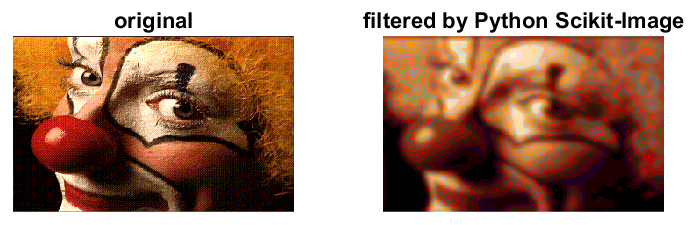## Matlab matrices to / from Python

Passing N-dimensional arrays to/from Python from Matlab is straightforward with Matlab. Matlab understands list(), dict(), set(), and other Python classes including Numpy ndarray and Xarray.

Matlab using Numpy: image_proc.m:Filter an image in Python and display in Matlab

Matlab using Xarray: see example glow.m.

``````function M = xarray2mat(V)
M = double(py.numpy.asfortranarray(V));
end

function I = xarrayind2vector(V,key)
% convert xarray index to Matlab 1-D vector

I = cell2mat(cell(V.indexes{key}.values.tolist));

end
``````

Related: